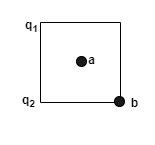# Problem: Point charges q1 = +2.00 μC and q2 = -2.00 μC are placed at adjacent corners of a square for which the length of each side is 3.50 cm. Point a is at the center of the square, and point b is at the empty corner closest to q2. Take the electric potential to be zero at a distance far from both charges.A) What is the electric potential at point a due to q1 and q2?B) What is the electric potential at point b?C) A point charge q3 = -5.00 μC moves from point a to point b. How much work is done on q3 by the electric forces exerted by q1 and q2?

###### FREE Expert Solution

Electric potential:

$\overline{){\mathbit{V}}{\mathbf{=}}\frac{\mathbf{kq}}{\mathbf{r}}}$(a)

r1a = r2a = (1/2)hypotenuse = (1/2)[(3.52 + 3.52)(1/2)] = 2.475cm(1m/100cm) = 0.02475m

97% (212 ratings)###### Problem Details

Point charges q1 = +2.00 μC and q2 = -2.00 μC are placed at adjacent corners of a square for which the length of each side is 3.50 cm. Point a is at the center of the square, and point b is at the empty corner closest to q2. Take the electric potential to be zero at a distance far from both charges.

A) What is the electric potential at point a due to q1 and q2?

B) What is the electric potential at point b?

C) A point charge q3 = -5.00 μC moves from point a to point b. How much work is done on q3 by the electric forces exerted by q1 and q2?